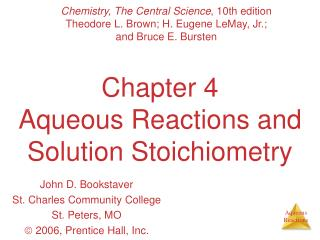DownloadDownload PresentationChapter 4 Aqueous Reactions and Solution Stoichiometry

# Chapter 4 Aqueous Reactions and Solution Stoichiometry

Download Presentation## Chapter 4 Aqueous Reactions and Solution Stoichiometry

- - - - - - - - - - - - - - - - - - - - - - - - - - - E N D - - - - - - - - - - - - - - - - - - - - - - - - - - -
##### Presentation Transcript

1. Chemistry, The Central Science, 10th edition Theodore L. Brown; H. Eugene LeMay, Jr.; and Bruce E. Bursten Chapter 4Aqueous Reactions and Solution Stoichiometry John D. Bookstaver St. Charles Community College St. Peters, MO  2006, Prentice Hall, Inc.

2. Electrolytes • Substances that dissociate into ions when dissolved in water. • Anonelectrolyte may dissolve in water, but it does not dissociate into ions when it does so.

3. Electrolytes and Nonelectrolytes Soluble ionic compounds tend to be electrolytes.

4. Electrolytes and Nonelectrolytes Molecular compounds tend to be nonelectrolytes, except for acids and bases.

5. Electrolytes • A strong electrolyte dissociates completely when dissolved in water. • A weak electrolyte only dissociates partially when dissolved in water.

6. Strong Electrolytes Are… • Strong acids • Strong bases • Soluble ionic salts

7. Precipitation Reactions When one mixes ions that form compounds that are insoluble (as could be predicted by the solubility guidelines), a precipitate is formed.

8. Metathesis comes from a Greek word that means “to transpose” It appears the ions in the reactant compounds exchange, or transpose, ions AgNO3 (aq) + KCl (aq)  AgCl (s) + KNO3 (aq) Metathesis (Exchange) Reactions

9. The molecular equation lists the reactants and products in their molecular form. AgNO3 (aq) + KCl(aq) AgCl(s) + KNO3 (aq) Molecular Equation

10. To form the net ionic equation, cross out anything that does not change from the left side of the equation to the right. The only things left in the equation are those things that change (i.e., react) during the course of the reaction. Those things that didn’t change (and were deleted from the net ionic equation) are called spectator ions. Ag+(aq) + NO3-(aq) + K+(aq) + Cl-(aq) AgCl(s) + K+(aq) + NO3-(aq) Net Ionic Equation

11. Writing Net Ionic Equations • Write a balanced molecular equation. • Dissociate all strong electrolytes. • Cross out anything that remains unchanged from the left side to the right side of the equation. • Write the net ionic equation with the species that remain.

12. Acids There are only seven strong acids: • Hydrochloric (HCl) • Hydrobromic (HBr) • Hydroiodic (HI) • Nitric (HNO3) • Sulfuric (H2SO4) • Chloric (HClO3) • Perchloric (HClO4)

13. Bases The strong bases are the soluble salts of hydroxide ion: • Alkali metals • Calcium • Strontium • Barium

14. When a strong acid reacts with a strong base, the net ionic equation is… HCl (aq) + NaOH (aq)  NaCl (aq) + H2O (l) H+ (aq)+ Cl- (aq)+ Na+ (aq) + OH-(aq) Na+ (aq)+ Cl- (aq)+ H2O (l) H+ (aq)+ Cl- (aq)+ Na+ (aq) + OH- (aq) Na+ (aq) + Cl- (aq) + H2O (l) Neutralization Reactions

15. Neutralization Reactions Observe the reaction between Milk of Magnesia, Mg(OH)2, and HCl.

16. Oxidation-Reduction Reactions • An oxidation occurs when an atom or ion loses electrons. • A reduction occurs when an atom or ion gains electrons.

17. Oxidation-Reduction Reactions One cannot occur without the other.

18. Oxidation Numbers To determine if an oxidation-reduction reaction has occurred, we assign an oxidation number to each element in a neutral compound or charged entity.

19. Oxidation Numbers • Elements in their elemental form have an oxidation number of 0. • The oxidation number of a monatomic ion is the same as its charge.

20. Oxidation Numbers • Nonmetals tend to have negative oxidation numbers, although some are positive in certain compounds or ions. • Oxygen has an oxidation number of −2, except in the peroxide ion in which it has an oxidation number of −1. • Hydrogen is −1 when bonded to a metal, +1 when bonded to a nonmetal.

21. Oxidation Numbers • Nonmetals tend to have negative oxidation numbers, although some are positive in certain compounds or ions. • Fluorine always has an oxidation number of −1. • The other halogens have an oxidation number of −1 when they are negative; they can have positive oxidation numbers, however, most notably in oxyanions.

22. Oxidation Numbers • The sum of the oxidation numbers in a neutral compound is 0. • The sum of the oxidation numbers in a polyatomic ion is the charge on the ion.

23. Displacement Reactions In this reaction, silver ions oxidize copper metal. Cu (s) + 2 Ag+ (aq) Cu2+ (aq) + 2 Ag (s)

24. Displacement Reactions The reverse reaction, however, does not occur. Cu2+ (aq) + 2 Ag (s)  Cu (s) + 2 Ag+ (aq) x

25. Activity Series

26. Two solutions can contain the same compounds but be quite different because the proportions of those compounds are different. Molarity is one way to measure the concentration of a solution. moles of solute Molarity (M) = volume of solution in liters Molarity

27. Using Molarities inStoichiometric Calculations

28. Titration The analytical technique in which one can calculate the concentration of a solute in a solution.

29. Titration